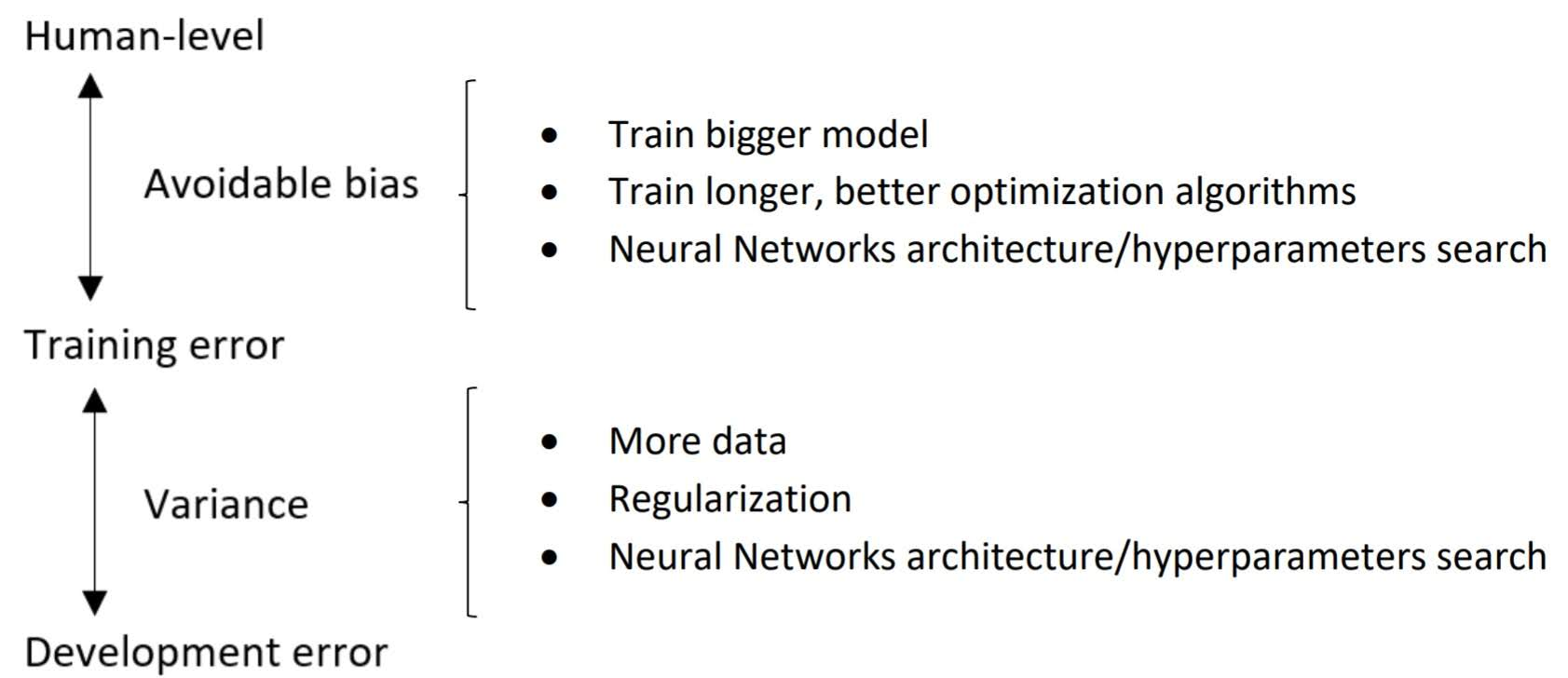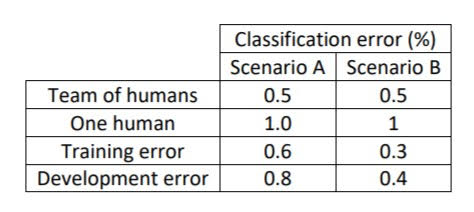Categories:

Updated:

# Bayes Optimal Error and Human-Level Error

Bayes optimal error is the smallest possible error that the algorithm can reach.

For some cases, you can estimate bayes optimal error with human-level error. For example in computer vision task like recognizing a cat, if error rate of human is 0.01%, we can say that bayes optimal error is about 0.01% or less.

For other cases where machines do a lot better than human, it is hard to estimate bayes optimal error. For example, in product recommendation task, machines have much less error rate than human, so we do not know how much more a algorithm can improve.

# Avoidable Bias and Variance

‘Avoidable bias’ = ‘training error’ - ‘bayes optimal error’

‘Variance’ = ‘development error’ - ‘training error’

However, nobody knows exact value of bayes optimal error in any task. So we usually use human-level error instead of bayes optimal error when diagnosing how well a model is doing.

Let’s say that a model’s human-level error is 5%, training error is 10% and development error is 11%. Then avoidable bias is 5% point and variance is 1% point. Avoidable bias is larger than variance, thus we should try reducing bias.
We may try;

• train bigger model
• train longer
• use better optimization algorithms
• regularize less

When avoidable bias is smaller than variance, we should try reducing variance.
We may try;

• train with more data
• regularize more
• use dropout
• train smaller model# After surpassing human level performance

When our algorithm surpass human level performance, it is difficult to diagnose which way to prioritize.For example, in ‘Scenario B’ in the above table, training error and development error both are less than humen-level error. We know that variance is 0.1% point, but we do not know how much is avoidable bias.

Categories: